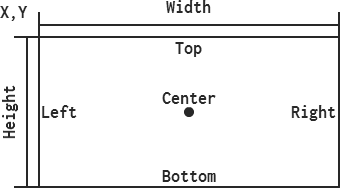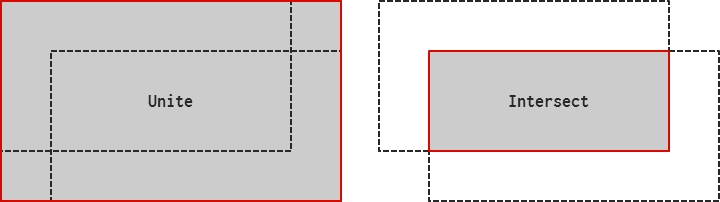# Bounds

Edit on GitHub
#include <Bounds.h>
SReturnNameParameters
Bounds (int32 value = 0)
Bounds (int32 x, int32 y, int32 w, int32 h)
Bounds (const Point& p, const Size& s)
bool IsZero (void) const
bool IsEmpty (void) const
bool IsValid (void) const
int32 GetLeft (void) const
int32 GetTop (void) const
int32 GetRight (void) const
int32 GetBottom (void) const
void SetLeft (int32 l)
void SetTop (int32 t)
void SetRight (int32 r)
void SetBottom (int32 b)
void GetLTRB (int32& l, int32& t, int32& r, int32& b)
void SetLTRB (int32 l, int32 t, int32 r, int32 b)
void Normalize (void)
bool Contains (const Point& p, bool inclusive = true) const
bool Contains (int32 x, int32 y, bool inclusive = true) const
bool Contains (const Bounds& b, bool inclusive = true) const
bool Contains (int32 x, int32 y, int32 w, int32 h, bool inclusive = true) const
bool Intersects (const Bounds& b, bool inclusive = true) const
bool Intersects (int32 x, int32 y, int32 w, int32 h, bool inclusive = true) const
Point GetPoint (void) const
void SetPoint (const Point& p)
void SetPoint (int32 x, int32 y)
Size GetSize (void) const
void SetSize (const Size& s)
void SetSize (int32 w, int32 h)
Point GetCenter (void) const
Bounds& operator |= (const Bounds& bounds)
Bounds& operator &= (const Bounds& bounds)
Bounds operator | (const Bounds& bounds) const
Bounds operator & (const Bounds& bounds) const
bool operator == (const Bounds& bounds) const
bool operator != (const Bounds& bounds) const
int32 X
int32 Y
int32 W
int32 H

## Description

Represents a rectangle defined by X, Y, W and H with integer precision. Each component can be manipulated directly through the exposed property values. X and Y represent the top-left corner of the bounds while W and H represent the width and height of the bounds, which could be negative. Calling Normalize swaps the left and right or top and bottom edges of the bounds, as needed, to make the size positive.The GetLTRB and SetLTRB functions provide an alternative way for manipulating the bounds. These functions express bounds in much more traditional fashion by using left, top, right, and bottom to represent each of the four edges of the bounds. Functions pertaining to left and right modify the X and Width components while top and bottom modify the Y and Height components.

The IsZero function returns true if all components are equal to zero while the IsEmpty function returns true if either Width or Height are equal to zero. An additional function, IsValid, returns true if both Width and Height are greater than zero, and the GetCenter function returns a point at the center of the bounds.

The Bounds class also offers an additional set of functionality for testing and manipulating the geometry of the bounds. For instance, the Contains function checks whether a point is inside or on the edges of the bounds. The other Contains function performs the same task but with a bounds object instead of a point. Moreover, a bounds can be passed to the Intersects function which checks whether any part of it is within or on the edges of the bounds. Two bounds can be united or intersected through special overloaded operators.## Constructors

```

Bounds
(int32 value = 0)

Bounds
(int32 x, int32 y,
int32 w, int32 h)

```

Constructs a bounds with all components set to value or x, y, w, and h.

```

Bounds
(const Point& p,
const  Size& s)

```

Constructs a bounds with position set to p and size set to s.

## Functions

```

bool
IsZero
(void) const

```

Returns true if all components are equal to zero.

```

bool
IsEmpty
(void) const

```

Returns true if either Width or Height are equal to zero.

```

bool
IsValid
(void) const

```

Returns true if both Width and Height are greater than zero.

```

int32
GetLeft
(void) const

int32
GetTop
(void) const

int32
GetRight
(void) const

int32
GetBottom
(void) const

```

Returns the left, top, right, and bottom edges of this bounds.

```

void
SetLeft
(int32 l)

void
SetTop
(int32 t)

void
SetRight
(int32 r)

void
SetBottom
(int32 b)

```

Sets the left, top, right, and bottom edges of this bounds to l, t, r, and b.

```

void
GetLTRB
(int32& l, int32& t,
int32& r, int32& b)

```

Sets l, t, r, and b to the left, top, right, and bottom edges of this bounds.

```

void
SetLTRB
(int32  l, int32  t,
int32  r, int32  b)

```

Sets the left, top, right, and bottom edges of this bounds to l, t, r, and b.

```

void
Normalize
(void)

```

Normalizes this bounds to ensure that Width and Height are positive. If Width is negative, this function swaps the left and right edges. If Height is negative, this function swaps the top and bottom edges.

```

bool
Contains
(const Point& p,
bool inclusive = true) const

bool
Contains
(int32 x, int32 y,
bool inclusive = true) const

```

Returns true if p is within or on the edges of this bounds. If inclusive is false, this function returns true only if p is entirely within this bounds and not on the edges. This function works even when Width and Height are negative.

```

bool
Contains
(const Bounds& b,
bool inclusive = true) const

bool
Contains
(int32 x, int32 y,
int32 w, int32 h,
bool inclusive = true) const

```

Returns true if b is within or on the edges of this bounds. If inclusive is false, this function returns true only if b is entirely within this bounds and not on the edges. This function works even when Width and Height are negative.

```

bool
Intersects
(const Bounds& b,
bool inclusive = true) const

bool
Intersects
(int32 x, int32 y,
int32 w, int32 h,
bool inclusive = true) const

```

Returns true if any part of b intersects with this bounds. If inclusive is false, this function returns true only if any part of b is entirely within this bounds and not on the edges. This function works even when Width and Height are negative.

```

Point
GetPoint
(void) const

```

Returns a Point with this bounds X and Y components.

```

void
SetPoint
(const    Point& p)

void
SetPoint
(int32 x, int32  y)

```

Sets the X and Y components of this bounds to p.

```

Size
GetSize
(void) const

```

Returns a Size with this bounds Width and Height components.

```

void
SetSize
(const    Size& s)

void
SetSize
(int32 w, int32 h)

```

Sets the Width and Height components of this bounds to s.

```

Point
GetCenter
(void) const

```

Returns a Point representing the center of this bounds.

## Operators

```

Bounds&
operator |=
(const Bounds& bounds)

```

Unites bounds with this bounds and returns a reference to this bounds. This function works even when Width and Height are negative and always generates a normalized bounds.

``````Bounds b1 (0, 0, 4, 4);
Bounds b2 (2, 2, 4, 4);

// b1 becomes (0, 0, 6, 6)
b1 |= b2;
``````

```

Bounds&
operator &=
(const Bounds& bounds)

```

Intersects bounds with this bounds and returns a reference to this bounds. If there is no intersection, a default bounds is returned. This function works even when Width and Height are negative and always generates a normalized bounds.

``````Bounds b1 (0, 0, 4, 4);
Bounds b2 (2, 2, 4, 4);

// b1 becomes (2, 2, 2, 2)
b1 &= b2;
``````

```

Bounds
operator |
(const Bounds& bounds) const

```

Unites bounds with this bounds and returns the result in a new bounds. This function works even when Width and Height are negative and always returns a normalized bounds.

```

Bounds
operator &
(const Bounds& bounds) const

```

Intersects bounds with this bounds and returns the result in a new bounds. If there is no intersection, a default bounds is returned. This function works even when Width and Height are negative and always returns a normalized bounds.

```

bool
operator ==
(const Bounds& bounds) const

bool
operator !=
(const Bounds& bounds) const

```

Performs equality comparison on each component.

## Properties

```

int32
X

int32
Y

int32
W

int32
H

```

Provides direct access to the X, Y, Width and Height components of this bounds.

# Examples

``````// C++
#include <Robot.h>
ROBOT_NS_USE_ALL;

int main (void)
{
Bounds b1, b2 (2, 4, 8, 6);

b1.IsZero (); // True
b1.IsEmpty(); // True
b1.IsValid(); // False
b2.IsValid(); // True

// b1 becomes (2, 4, 8, 6)
b1.SetPoint (2, 4);
b1.SetSize  (8, 6);

// Returns (6, 7)
b1.GetCenter();

b1 == b2; // True
b1 != b2; // False

// b1 becomes (6, 7, -6, -5)
b1.SetLTRB (6, 7, 0, 2);
// b1 becomes (0, 2,  6,  5)
b1.Normalize();

b1.Contains (5, 5); // True
b1.Contains (6, 6); // True
b1.Contains (5, 5, false); // True
b1.Contains (6, 6, false); // False

b1.Contains   (b2); // False
b1.Intersects (b2); // True

// b3 becomes (0, 2, 10, 8)
Bounds b3 = b1 | b2;
// b4 becomes (2, 4,  4, 3)
Bounds b4 = b1 & b2;
return 0;
}
``````
``````// Node
var robot = require ("robot-js");

var b1 = robot.Bounds ();
var b2 = robot.Bounds (2, 4, 8, 6);

b1.isZero (); // True
b1.isEmpty(); // True
b1.isValid(); // False
b2.isValid(); // True

// b1 becomes (2, 4, 8, 6)
b1.setPoint (2, 4);
b1.setSize  (8, 6);

// Returns (6, 7)
b1.getCenter();

b1.eq (b2); // True
b1.ne (b2); // False

// b1 becomes (6, 7, -6, -5)
b1.setLTRB (6, 7, 0, 2);
// b1 becomes (0, 2,  6,  5)
b1.normalize();

b1.containsP (5, 5); // True
b1.containsP (6, 6); // True
b1.containsP (5, 5, false); // True
b1.containsP (6, 6, false); // False

b1.containsB  (b2); // False
b1.intersects (b2); // True

// b3 becomes (0, 2, 10, 8)
var b3 = b1.unite (b2);
// b4 becomes (2, 4,  4, 3)
var b4 = b1.intersect (b2);

// Bounds construction
b1 = robot.Bounds (b2);

b1 = robot.Bounds
(
robot.Point (100, 200),
robot.Size  (300, 400)
);

b1 = robot.Bounds
({
x: 100, y: 200,
w: 300, h: 400
});

b1 = robot.Bounds
({
l: 100, t: 200,
r: 300, b: 400
});

b1 = robot.Bounds
(
{ x: 100, y: 200 },
{ w: 300, h: 400 }
);
``````